## Large-scale correlation analysis with the hyperGroup CAS Action

Started ‎12-16-2019 by
Modified ‎12-16-2019 by
Views 3,399

A fundamental task for statisticians, data scientists and other analysts: Determine if thousandseven millionsof variables change together in a meaningful way. Fast, accurate large-scale correlation analysis is the how. Your business need is the why.

You care about it if, for example, you're portfolio manager trying to correlate the values of thousands of stocks, bonds, mutual funds, commodities, etc., using data about them at many points in time. You'd isolate and visualize communities of values. When some in a community are going up (or down), you may buy the ones likely to rise (or fall). The decision depends on whether they're comparatively under- or over-valued. You may even want to buy or sell assets known to move in opposite directions.

This article details how to use SAS Viya's hyperGroup CAS action for such a project. At the end, you'll find links to documentation, resources on correlation matrices, graphs, communities of variables and more.

Consider the correlation matrix pictured below. It has rows and columns in the same but random order. Only the correlations that aren't weak are shown. You choose what is "not weak" – it could be correlation > 0.3 or correlation < -0.3, for instance. In some cases, filtering is unnecessary as the correlation matrix is already sparse.Figure 1. A noisy correlation matrix

This big, noisy matrix doesn't allow you to glean much insight. Upon zooming in, you might learn how a variable is correlated to another. By examining what's in a column downwards from a variable's diagonal and in a row across to that diagonal, you might discern how a variable is correlated to all the others. Only so much information can be learnt as had the matrix been small.

Incidentally, this matrix is 5,000 x 5,000 and has about 25,000 elements – i.e. there are 5,000 variables and 25,000 strong distinct correlations between them.

After calculating correlations, you need to:

1. Determine communities of variables.
2. Order communities in a sensible manner.
3. Determine how variables in each community should be ordered.
4. Design visual results that you can view, gain insight, and make decisions.

(Editor's note: If you want a separate explanatory post on the above four steps, including graphs of correlations and 2D and 3D structural graphs, comment below.)

Graphs

To most people, "graph" is synonymous with "plot". Here, it has an entirely different and precise mathematical description, that is: G = (V,E), where V is a set of vertices (singular tense is vertex), and E is a set of edges so that each edge is defined by the two vertices it connects. For each edge, its vertices are said to be adjacent. A graph can be defined by an adjacency matrix A where A[i,j]=1 if vertices i and j are adjacent, and 0 otherwise. A is symmetric, as we assume edges have no direction.

In this setting, we work on a graph that has the same nonzero elements in A as does the correlation matrix C, i.e. A[i,j]=1 if C[i,j]!=0, and 0 otherwise. Therefore, we use interchangeably vertex and variable (vertices and variables), and we use interchangeably edge for correlation.

Here is a graph (a smaller example with similar characteristics) for the above correlation matrix:1. Determine communities of variables

From the above graph and how vertices are colored, you probably already have a sense what communities are: each vertex belongs to the same community as do the majority of vertices adjacent to it... except there are subtle catch- correlation values are taken into account.

Consider this little graph and its adjacency matrix (only the lower triangular part needed):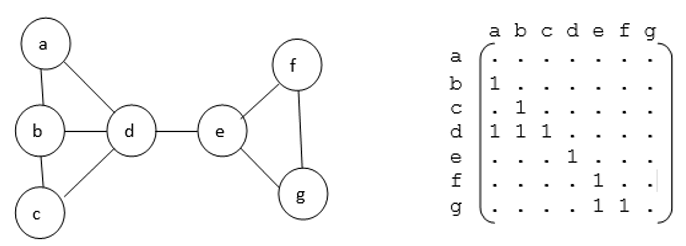It looks as if community 1 should be {a,b,c,d} and community 2 should be {e,f,g}. But imagine that C[d,e]= -0.9, C[e,f]=0.5, and C[e,g]= -0.3. In this case, the algorithm that determines correlation communities would put e into community 1, as the sum of absolute values of correlations of edges between e and community 1 vertices exceeds the sum of absolute values of correlations of edges between e and community 2 vertices, i.e.

abs(C[d,e]) > abs(C[e,f]) + abs(C[e,g])

0.9      >       0.5        +       0.3

1. Order communities

Before describing how communities are ordered, we define a structural graph as having a vertex for each community, and edges such that there is one edge between communities i and j if at least any variable in community i is connected/correlated to any variable in community j.

Here's the structural graph for our example, with actual variables shown:The weight of an edge (i,j) in the structural graph is the sum of the absolute correlations when one variable is in community i and the other variable is in community j.

The algorithm hyperGroup uses strives to "keep close" communities that have weighty inter-community correlations in common, and to "have separation" between communities that have less weighty if any such correlations in common. All correlations should be close to the diagonal.

## How the hyperGroup CAS actionworks

With SAS Viya's hyperGroup CAS action, part ofhyperGroup CAS action set, start by extracting elements from the correlation matrix to create a CAS table corrMatrix, that has three columns: x, y, and corr. That table has a record for each correlation ( i,j,C[i,j), when C[i,j] is not close to 0.0, or all correlations if the correlation matrix is sparse from the outset. Then run:

``````s:hyperGroup_hyperGroup{indexC=True,absFreq=True,
community=true,structural="COMMUNITY",
nocolor=true,createOut="NEVER",graphPartition=true,maxnodes=150.0,
table    ={name="corrMatrix"},
inputs   ={{name="x"},{name="y"}},
frequency={"corr"},
vertices ={name="verticesout", replace=true},
edges    ={name="edgesout",    replace=true},
edges3   ={name="ECommStrlout",replace=true},
vertices3={name="VCommStrlout",replace=true}}  ``````

### Breaking down the code

• indexC=True, specifies you want the variables indexed, considering which variables are in each community, and how the communities are ordered.

A strange quirk you may notice above is that the correlation information in the corr column is specified in the frequency variable list. HyperGroup was originally written to, among other things, conduct Social Network Analysis when the data was for how people are connected – and by how many times – their frequency.

• absFreq=True, specifies that absolute values of correlations are used by the community detection algorithm.
• The output tables vertices= and edges= contain information about the variables and edges of the correlations.
• The output tables vertices3= and edges3= contain information about the structural graph.
• Note: indexC and absFreq are new options that will be available in the version of hyperGroup slated for an upcoming release.

Contents of the output tables, especially coordinates of vertices, are needed by graph renderers.

Of greatest interest to correlation analysis is contained in the edges= output table. There is one record for each correlation. Correlations are in the _Frequency_ column. Variables are in the _Source_ and _Target_ columns, and the index of the variables after communities are ordered are in _SindexC_ and _TindexC_, respectively

This code:

``````data permutated;
set cas.edgesout;
if _SindexC_ > _TindexC_ then do;  /* reflect back into lower triangular */
i=_SindexC_; _SindexC_=_TindexC_; _TindexC_=i;
end;
drop i;
run;

title "correlation matrix, permutated";
proc sgplot data=permutated;
scatter x=_SindexC_ y=_TindexC_/markerattrs=(symbol=CircleFilled size=2);
yaxis reverse;
run; ``````

produces this plot: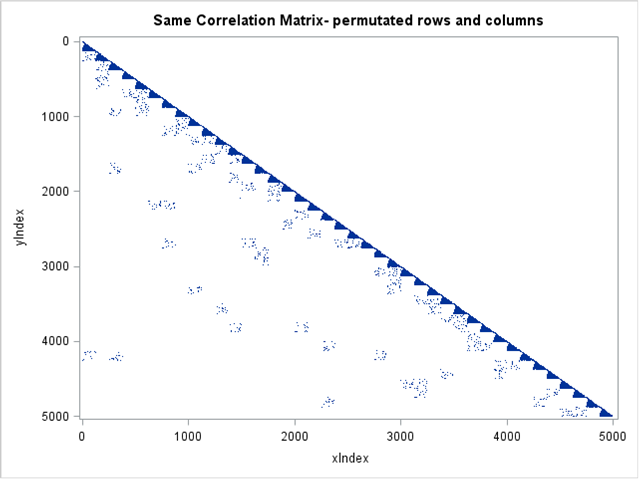Figure 2. Permutated rows and columns

Below, the matrix on the right is the same matrix as on the left whose rows and columns have been symmetrically permutated: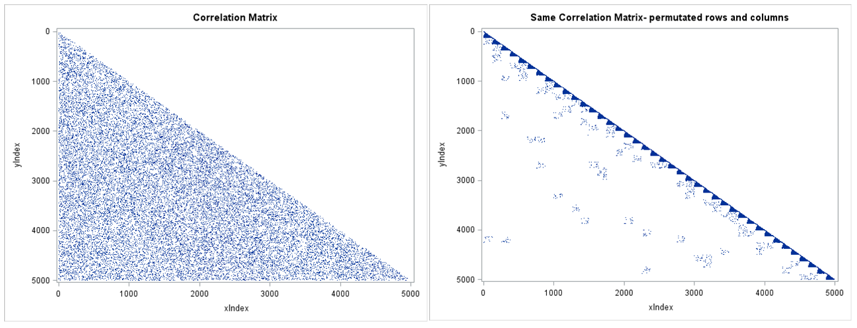Figure 3.

• The vertices= output table has a record for each variable. Columns contain the name of variables, the index of the variable after communities are ordered, the coordinates of the associated vertex, and the community to which the variable belongs.
• The edges3= output table has a record for each structural graph edge. The columns contain the communities the edge connects (i and j say), the number of correlations between variables in community i and variables in community j, and the sum of absolute values of correlations of those inter-community edges.
• The vertices3= output table has a record for structural graph vertex, i.e. each community. Columns contain the community number, coordinates, the center of mass of the community with respect to variable vertices locations, the number of variables in each community, the number of edges that connect them, and the sum of absolute values of correlations of those intra-community edges.

## Hypergroupsreveal which variables matter

The hyperGroup CAS action may determine that there are sets of variables that have no connection to other sets of variables, with respect to their correlations.

To illustrate this property, I replicated the data three times, thus artificially creating new data that has three hypergroups. After running hyperGroup, output tables all have a _HypGrp_ column with values 0, 1, or 2 indicating which hypergroup the record of, depending on the output table, the vertex, edge, structural graph vertex, or structural graph edge belongs, and producing the permutated correlation matrix (that now has 15,000 variables, about 70,000 correlations) like Figure 2, we obtain: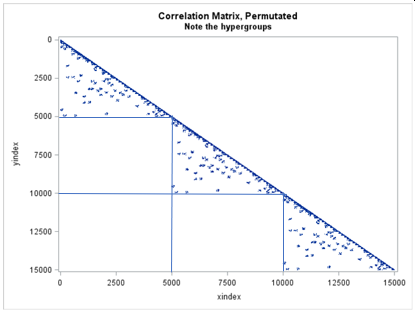Figure 4.

## Visualizations to aid your understanding, some in 3D

So far, we have shown graphs of correlations and structural graphs in 2D, but the CAS action that does all the work can do so in 3DBelow are examples of 3D graphs determined by the CAS action, using various ways to render them, such as SAS and JMP, some commonly used libraries available in Python, and Unity3d, the latter allowing use of virtual reality, which is extremely immersive.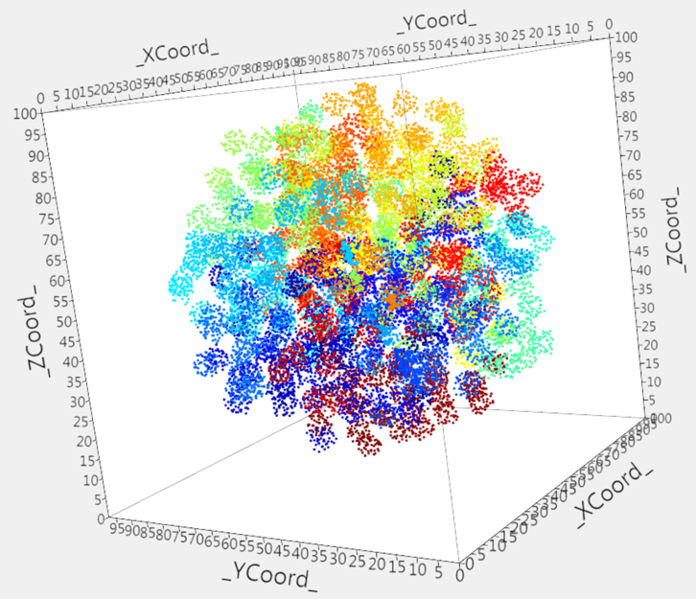SASJMP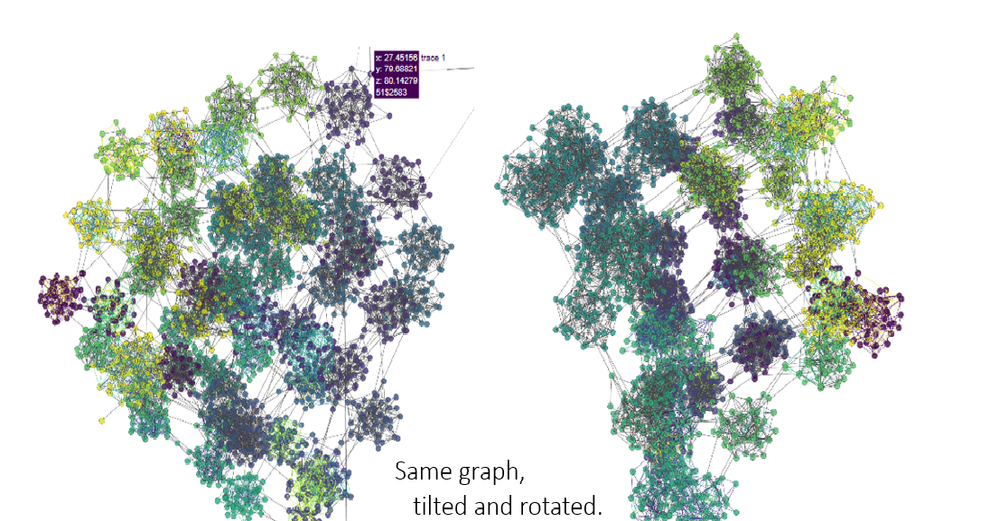Plotly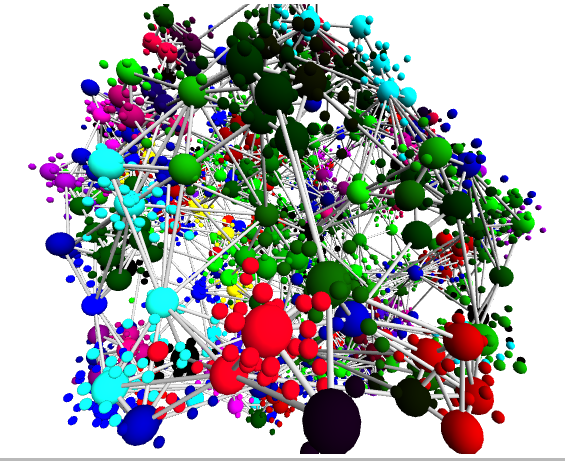jgraph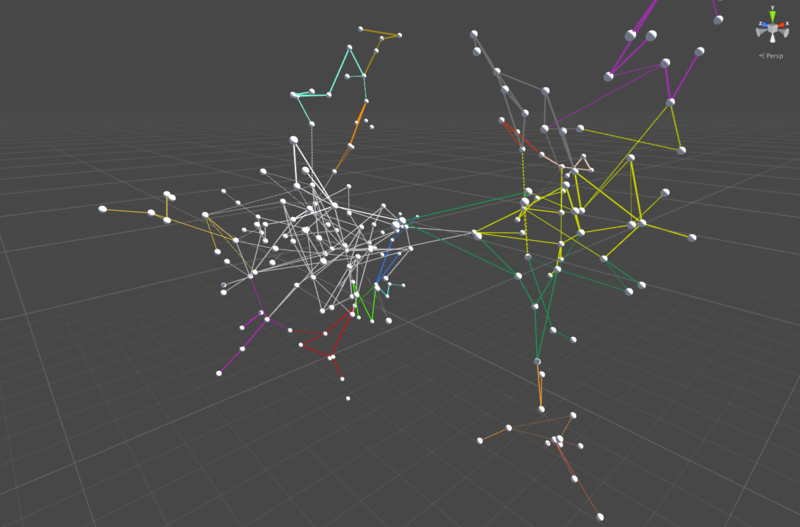Unity

Most renderer systems allow you can tilt and rotate, pan, zoom- the vertices/variables fly around in formation like well-practiced aerobatic teams. You program what is displayed when you hover above vertices and edges. You have considerable control over appearance aspects, such as sizes of vertices, colors, icon shapes, etc. You can change your vantage point around and within graphs to see data from unimagined perspectives.

It never ceases to amaze how data that seems featureless hides beautiful structure and associations.

## Conclusion

By combining correlation analysis (usually taught in statistics) with graph theory (usually taught as part of operations research), we learn how a great many variables behave together and are placed in communities. Somethough they seem to behave togetherbelong in different communities.

WitSAS Viya's hyperGroup CAS action, crucial computations to analyze correlations are quick and easy, even those involving thousands of variables. The data may be even greater in scale, yet remain well within the capabilities of the softwareso that visualizations result in sound business decisions.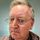Trever, some of the visualisations do indeed look suitable for the Virtual Reality treatment!

Point me at some data, and I'll render them in the VR software, and upload a video.

If that makes sense?

Version history
Last update:
‎12-16-2019 03:14 PM
Updated by:Contributors
Article Labels
Article Tags